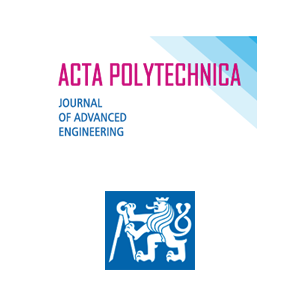Tetiana Kresan, Serhii Pylypaka, Zynovii Ruzhylo, Ivan Rogovskii, Oleksandra Trokhaniak

# External rolling of a polygon on closed curvilinear profile

Číslo: 4/2020
Periodikum: Acta Polytechnica
DOI: 10.14311/AP.2020.60.0313

Klíčová slova: Equilateral polygon, curvilinear profile, external rolling, differential equation, centroids

Pro získání musíte mít účet v Citace PRO.

Anotace: The rolling of a flat figure in the form of an equilateral polygon on a curvilinear profile is considered. The profile is periodic. It is formed by a series connection of an arc of a symmetrical curve. The ends of the arc rely on a circle of a given radius. The equation of the curve, from which the curvilinear profile is constructed, is found. This is done provided that the centre of the polygon, when it rolls in profile, must also move in a circle. Rolling occurs in the absence of sliding. Therefore, the length of the arc of the curve is equal to the length of the side of the polygon. To find the equations of the curve of the profile, a first-order differential equation is constructed. Its analytical solution is obtained. The parametric equations of the curve are obtained in the polar coordinate system. The limits of the change of an angular parameter for the construction of a profile element are found. It is a part of the arc of the curve. According to the obtained equations, curvilinear profiles with different numbers of their elements are constructed.